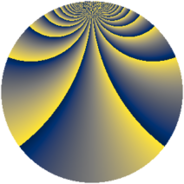# Properties

 Label 2300.2.biLevel $2300$ Weight $2$ Character orbit 2300.bi Rep. character $\chi_{2300}(243,\cdot)$ Character field $\Q(\zeta_{44})$ Dimension $4240$ Sturm bound $720$

# Related objects

## Defining parameters

 Level: $$N$$ $$=$$ $$2300 = 2^{2} \cdot 5^{2} \cdot 23$$ Weight: $$k$$ $$=$$ $$2$$ Character orbit: $$[\chi]$$ $$=$$ 2300.bi (of order $$44$$ and degree $$20$$) Character conductor: $$\operatorname{cond}(\chi)$$ $$=$$ $$460$$ Character field: $$\Q(\zeta_{44})$$ Sturm bound: $$720$$

## Dimensions

The following table gives the dimensions of various subspaces of $$M_{2}(2300, [\chi])$$.

Total New Old
Modular forms 7440 4400 3040
Cusp forms 6960 4240 2720
Eisenstein series 480 160 320

## Trace form

 $$4240q + 18q^{2} - 20q^{6} + 18q^{8} + O(q^{10})$$ $$4240q + 18q^{2} - 20q^{6} + 18q^{8} + 6q^{12} + 36q^{13} - 20q^{16} + 36q^{17} + 38q^{18} - 40q^{21} + 28q^{22} - 36q^{26} + 34q^{28} - 2q^{32} + 60q^{33} - 132q^{36} + 36q^{37} + 10q^{38} - 104q^{41} + 202q^{42} - 92q^{46} + 50q^{48} + 250q^{52} + 84q^{53} - 60q^{56} + 44q^{57} + 58q^{58} + 56q^{61} + 82q^{62} - 84q^{66} + 4q^{68} + 104q^{72} + 36q^{73} - 20q^{76} + 44q^{77} + 50q^{78} + 128q^{81} + 10q^{82} - 76q^{86} + 50q^{88} + 22q^{92} + 88q^{93} + 28q^{96} + 36q^{97} - 30q^{98} + O(q^{100})$$

## Decomposition of $$S_{2}^{\mathrm{new}}(2300, [\chi])$$ into newform subspaces

The newforms in this space have not yet been added to the LMFDB.

## Decomposition of $$S_{2}^{\mathrm{old}}(2300, [\chi])$$ into lower level spaces

$$S_{2}^{\mathrm{old}}(2300, [\chi]) \cong$$ $$S_{2}^{\mathrm{new}}(460, [\chi])$$$$^{\oplus 2}$$